# DAV Class 4 Maths Chapter 4 Brain Teasers Solutions

The DAV Class 4 Maths Book Solutions and DAV Class 4 Maths Chapter 4 Brain Teasers Solutions of Division offer comprehensive answers to textbook questions.

## DAV Class 4 Maths Ch 4 Brain Teasers Solutions

Question 1.
(a) There are _______ dozens in 264.
(i) 44
(ii) 22
(iii) 13
(iv) 11
(ii) 22

(b) 2 lakhs × _______ = 20 Lakhs
(i) 0
(ii) 10
(iii) 1
(iv) 100
(ii) 10

(c) 7 × 3 × 0 × 5 = _______
(i) 21
(ii) 15
(iii) 0
(iv) 105
(iii) 0

(d) If divisor = 7, Remainder = 3, Quotient = 3 then, Dividend =
(i) 13
(ii) 16
(iii) 21
(iv) 24
(iv) 24

(e) Product of the greatest 2-digit number and the smallest 3-digit number is _______
(i) 990
(ii) 9000
(iii) 99000
(iv) 9900
(iv) 9900 (99 × 100)Question 2.
Solve the following sums.
(a) 3,282 × 213(b) 19,816 × 6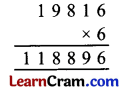(c) 4,172 ÷ 26Q = 160
R = 12

(d) 14,865 ÷ 15Q = 991
R = 0

Question 3.
Replace ★ by the correct number.
(a)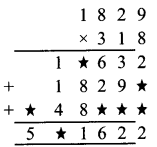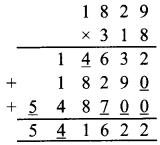(b) 432 ÷ ★ = 24(c) ★÷ 60 = 10
Let the number be x
x = 60 × 10
= 600

(d)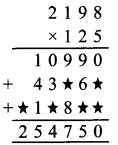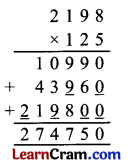Question 4.
(a) 2,000 by 12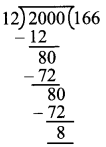Dividend = Divisor × Q + R
L.H.S. = 2000
R.H.S. = 12 × 166 + 8
= 1992 + 8
= 2000
∴ R.H.S = L.H.S

(b) 7682 by 45Dividend = Divisor × Q + R
L.H.S. = 7682
R.H.S. = 45 × 170 + 32
= 7650 + 32
= 7682
∴ R.H.S = L.H.S

Question 5.
Given that 270×15 = 4,050, find the product.
(a) 270 × 16
270 × 15 4,050
(a) 270 × 16
= 270 × (15 + 1) (using distributive property)
= 270 × 15 + 1 × 270
= 4050 + 270
= 4,320

(b) 270 × 14
270 × 14
270 × (15 – 1)
(using distributive property)
= 270 × 15 – 270 × 1
= 4050 – 270
= 3,780Question 6.
What is the total cost of fencing ₹ 275 plots of land if the cost of fencing one plot of land is 950?
1 plot has cost of fencing = ₹ 950
275 plots have cost of fencing = 950 × 275
= ₹ 2,61,250
Thus, total cost of fencing 275 plots of land is ₹ 2,61,250.

Question 7.
Fill in the blanks.
(a) 7,612 × ____ = 40 × 7,612
40

(b) ____ × 1 = 89,210
89,210

(c) 515 × ____ × 18 = 5 × ____ × 18
5, 515

(d) 10 × ____ = 8,000
800

(e) 176 ÷ ____ = 176
1

(f) ____ ÷ 82 = 0
0

(g) If 85,715 ÷ 35 = 2,449 then 85,715 ÷ 2,449 = ____
35

(h) 7,542 ÷ 100, quotient = ____ and remainder = ____
7, 542

Question 1.
Divide and find the quotient and remainder.
(i) 20566 by 99Q = 207
R = 63

(ii) 7708 by 28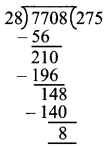Q = 275
R = 8

(iii) 9548 by 38Q = 251
R = 10

(iv) 9871 by 29Q = 340
R = 11

(v) 9025 by 85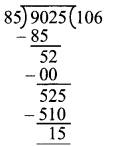Q = 106
R = 15Question 2.
Solve the following:
(i) dividend = 5940 and divisor = 54, find quotient.
(ii) dividend = 7560 and quotient = 63, find divisor.
Dividend = 5940, divisor = 54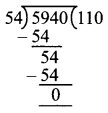Checking:
Dividend = Divisor × Q + R
R.H.S. =54 × 110 + 0
= 5940 + 0
= 5940
∴ R.H.S. = L.H.S.

(ii) Dividend = 7560 and quotient = 63
Dividend = Divisor × Q + R
7560 = Divisor × 63
$$\frac{7560}{63}$$ = Divisor
Divisor = 120Question 3.
Using the rule of division by 10, 100 and 1000. Find the quotient and the remainder for each of the following.
(a) 4807 ÷ 10
(b) 9436 ÷ 10
(c) 75311 ÷ 100
(d) 13560 ÷ 100
(e) 371351 ÷ 1000
(f) 473522 ÷ 1000
(a) 4807 ÷ 10
Q = 480
R = 7

(b) 9436 ÷ 10
Q = 943
R = 6

(c) 75311 ÷ 100
Q = 753
R = 11

(d) 13560 ÷ 100
Q = 135
R = 6

(e) 371351 ÷ 1000
Q = 371
R = 351

(f) 473522 ÷ 1000
Q = 473
R = 522

Question 4.
5 ,301 balls are packed equally in 93 boxes. How many balls are packed in each box?
Number of balls in 93 boxes = 5301
Number of balls in 1 box = 5301 ÷ 93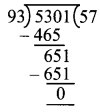Question 5.
The product of two numbers is 8855. If one of the number is 23. Find the other number.
Product of two numbers = 8855
one number = 23
∴ other number = 8855 ÷ 23
= 385Question 6.
Fill in the blanks:
(i) We start division from the extreme ______ of dividend.